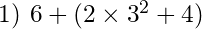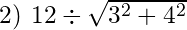# Confused by BIDMAS / BODMAS / BEDMAS / PEMDAS? Try GEMA!

## Remind me again, what’s BIDMAS?

If you grew up in the UK then it’s likely that you’ve been taught BIDMAS or BODMAS to help you remember the order of operations – what order you should do things in – in Maths. In other parts of the world, it’s BEDMAS or PEMDAS.

(In PEMDAS, P = Parentheses and E = Exponent.)

But BIDMAS and all the rest can be misleading, and it’s easy to get confused. The main reasons for confusion are:

• Some forms of notation count as “invisible brackets”
• e.g. in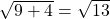, the roof of the root sign forms “invisible brackets”
• Division and multiplication have equal priority
• (They are flip sides of the same coin: dividing by 3 is the same as multiplying by, and vice versa)
• Addition and subtraction have equal priority
• (They are flip sides of the same coin: subtracting 2 is the same as adding –2, and vice versa)

When multiplying and dividing, or when adding and subtracting, we normally work from left to right; you DON’T do all the multiplying before the dividing, or all the addition before the subtraction, unless it’s written in that order.

## So what’s GEMA then?

An alternative acronym that you might find less confusing is GEMA:

First you work out any expressions that are grouped, for example:

• in brackets: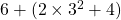• under a root sign: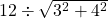• in the numerator or denominator of a fraction: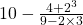– in this case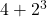is one grouping and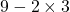is another.

Of course you will need to use the later steps within these groupings! Read on for some examples.

Exponent is another word for an index or power – which includes roots (since a root can be written as a power), e.g.

• with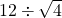you work out the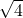first
• with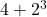you work out the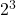first
• with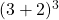you work out the 3 + 2 (the grouping) first and then cube it

With multiplication and division you just need to remember that the operator (x or ÷) belongs with the number immediately AFTER it. Generally it makes sense to work from left to right, but as long as you keep the operator with its number, the order doesn’t actually matter.

The same applies with addition and subtraction.

For example if you have 7 – 5 + 6 – 2 then you could do 7 take away 5, then add 6, then take away 2 (giving an answer of 6)…

OR

You could say, “We have positive 7 and positive 6, giving positive 13, and negative 5 and 2, giving negative 7, so overall we have +13 – 7 = 6.”

What you MUST NOT do – although BIDMAS might make you want to – is to say “Addition first, so we do 5 + 6 = 11, so that gives us 7 – 11 – 4, which is negative 8.”

## Give it a try!

Now have a go at these, using GEMA, and then watch the video to see if you got them right. Don’t just go straight to the video, try it yourself first!